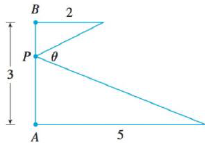Chapter 4.7, Problem 77E### Single Variable Calculus: Early Tr...

8th Edition
James Stewart
ISBN: 9781305270343

#### Solutions

Chapter
Section### Single Variable Calculus: Early Tr...

8th Edition
James Stewart
ISBN: 9781305270343
Textbook Problem

# Where should the point P be chosen on the line segment AB so as to maximize the angle θ?To determine

To find: The position of P on the line segment AB so that θ is maximum.

Explanation

Given:

Length of AB =3.

Calculation:

CPD=θ.

In Figure 1,

Let, BPC=α and APD=β.

Let BP=x.

Hence,

AP=3x.

In triangle ΔPBC,

tanα=2xα=tan1(2x)

tanβ=53xβ=tan1(53x)

Now,

θ+α+β=180θ=180αβ

Substitute α=tan1(2x) and β=tan1(53x) in θ,

θ=180tan1(2x)tan1(53x)

Differentiate θ with respect to x,

θ=(11+(2x)2×(2x2))(11+(53x)2×5(3x)2)=24+x25x26x+34

For critical points, θ=0

24+x25</

### Still sussing out bartleby?

Check out a sample textbook solution.

See a sample solution

#### The Solution to Your Study Problems

Bartleby provides explanations to thousands of textbook problems written by our experts, many with advanced degrees!

Get Started

#### Domain Find the domain of the expression. 11. x+3

Precalculus: Mathematics for Calculus (Standalone Book)

#### (x3y2z2)2

Applied Calculus for the Managerial, Life, and Social Sciences: A Brief Approach

#### True or False: converges if and only if .

Study Guide for Stewart's Multivariable Calculus, 8th

#### Evaluate dx using the Fundamental Theorem of Calculus. 6 12 18 24

Study Guide for Stewart's Single Variable Calculus: Early Transcendentals, 8th